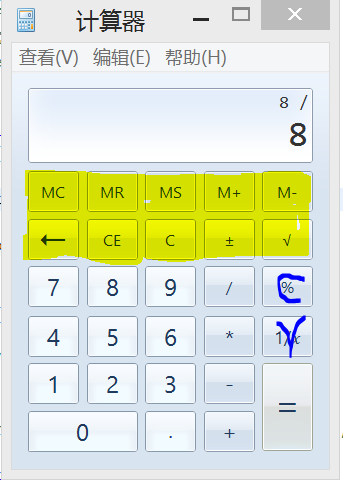java求解~~~大神帮帮忙import javax.swing.*;
import javax.lang.*;
import java.awt.*;
import java.awt.event.*;
import javax.swing.event.*;

class window1 extends JFrame {

String data[]={"1","2","3","4","5","6","7","8","9"};
JButton btn1[]=new JButton;
String op1[]={"+","-","*","/"};
JButton btn2[]=new JButton;
JButton reset=new JButton("C");
JButton clear=new JButton("CE");
JButton b1=new JButton("0");
JButton b2=new JButton(".");
JButton b3=new JButton("=");

JPanel sp = new JPanel();//显示框区域
JPanel bp=new JPanel();
JPanel p1=new JPanel();
JPanel p2=new JPanel();
JPanel p3=new JPanel();
JPanel p4=new JPanel();
JPanel p5=new JPanel();
JPanel p6=new JPanel();
JPanel p7=new JPanel();
int i;
JTextField sw_input=new JTextField(20);
JTextField sw_show=new JTextField(20);

window1(String s){

super(s);

setBackground(Color.LIGHT_GRAY);// 背景颜色
setBounds(500,500,345,480);
setVisible(true);
setDefaultCloseOperation(JFrame.EXIT_ON_CLOSE);
setResizable(false);// 不许修改计算器的大小
//setLocationRelativeTo(null);//居中显示
//pack();// 使计算器中各组件大小合适
setLayout(null);//设置空布局

sw_input.setEditable(false); // 不允许修改文本框
sw_input.setBackground(Color.WHITE);// 文本框背景颜色
//Panel calckeysPanel = new Panel();//计算器上键的按钮键在一个内

Font font_lab = new Font("arial", Font.BOLD, 22);//显示框布局
sw_input.setText("0");
sw_input.setHorizontalAlignment(JTextField.RIGHT); // 文本框右对齐
sw_input.setFont(font_lab);
font_lab = new Font("arial", Font.BOLD, 10);
sw_show.setText("sqrt");
sw_show.setHorizontalAlignment(JTextField.RIGHT); // 文本框右对齐
sw_show.setFont(font_lab);
sp.setBounds(14, 15, 300, 50);//设置显示框区域的位置的大小
sp.setLayout(null);//设置空布局
sp.setBorder(BorderFactory.createLineBorder(new Color(142,156,173)));//设置边框*/
sp.setLayout(new BorderLayout());
sp.setVisible(true);
/*Font font_but = new Font("arial", Font.BOLD, 10);//创建按钮字体
p1.setLayout(new GridLayout(4,1,6,6));//按键布局
p2.setLayout(new GridLayout(3,3,6,6));//按键布局
for(i=0;i<btn1.length;i++){
btn1[i]=new JButton(data[i]);
btn1[i].setPreferredSize(new Dimension(33,24));
btn1[i].setFont(font_but);//设置按钮字体
}
for(i=0;i<btn2.length;i++){
btn2[i]=new JButton(op1[i]);
btn2[i].setPreferredSize(new Dimension(33,24));
btn2[i].setFont(font_but);//设置按钮字体
btn2[i].setFont(font_but);//设置按钮字体
}
p3.setLayout(new BorderLayout());
b3.setPreferredSize(new Dimension(33,54));
p4.setLayout(new BorderLayout());
b1.setPreferredSize(new Dimension(72,24));
p5.setLayout(new BorderLayout());
bp.setLayout(new BorderLayout());
bp.setLayout(new BorderLayout());

}
}

public class jisuanqi3 {
public static void main(String args[]) {
window1 w=new window1("计算器");
}
}

3个回答